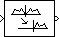# Analytic Signal

Compute analytic signals of discrete-time inputs

•Libraries:
DSP System Toolbox / Transforms

## Description

The Analytic Signal block computes the complex analytic signal y corresponding to each channel of the real input u.

`$y=u+jH\left\{u\right\},$`

where $j=\sqrt{-1}$ and H{ } denotes the Hilbert transform.

This block supports C/C++ code generation and SIMD code generation. For details, see Code Generation.

## Ports

### Input

expand all

Specify the data input u as a vector or a matrix of size M-by-N.

Data Types: `single` | `double`

### Output

expand all

Analytic signal output y, returned as a vector or a matrix.

The block computes the analytic signal for each channel. The real part of the output in each channel is a replica of the real input in that channel. The imaginary part of the output is the Hilbert transform of the input. In the frequency domain, the Fourier transform of the analytic signal doubles the positive frequency content of the original signal while zeroing out the negative frequencies and retaining the DC component.

The output has the same size and data type as the input.

Data Types: `single` | `double`
Complex Number Support: Yes

## Parameters

expand all

Specify the order of the equiripple FIR filter as an even positive integer. The FIR filter computes the Hilbert transform of the real input signal.

Specify how the block should process the input.

You can set this parameter to one of these options:

• `Columns as channels (frame based)` — The block performs frame-based processing. In this mode, the block treats an M-by-N matrix input as N independent channels containing M sequential time samples. The block computes the analytic signal for each channel over time.

• `Elements as channels (sample based)` — The block performs sample-based processing. In this mode, the block treats an M-by-N matrix input as M*N independent channels and computes the analytic signal for each channel (matrix element) over time.

## Block Characteristics

 Data Types `double` | `single` Multidimensional Signals `No` Variable-Size Signals `No`